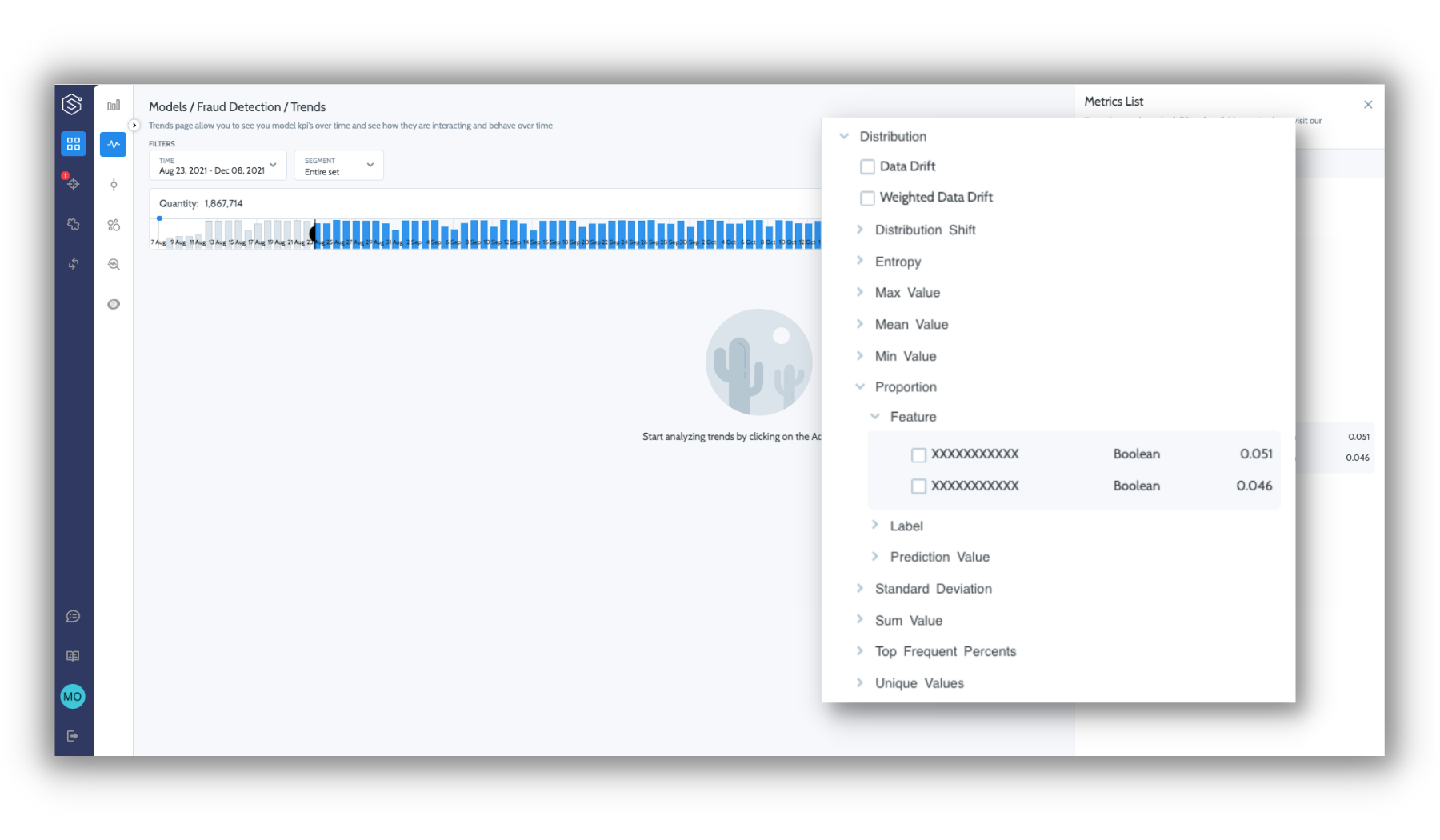# Distribution metrics

Statistical measures for any data entity throughout your ML processStatistical property metrics measured on the entity level are divided according to data entity format type:

MetricCategoricalNumericBoolean
Distribution shift
Top frequent percents
Frequency of the mode value.
--
Unique values
The number of unique values.
--
Entropy
Calculation of the entropy on the distribution of categorical entities.
--
Min value
The lowest value.
--
Max value
The highest value.
--
Sum value
The sum of values.
--
Mean value
The average value.
--
Standard Deviation
The measure of the amount of variation in a numeric entity.
--
Proportion
Percent of positive value.
--

#### Feature Importance

Superwise measures the effect a feature has on a model. Superwise calculates feature importance using SHAP values during version creation, using a reference dataset, or you can set this manually while configuring the schema.

#### Distribution shift & drift metrics:

• Distribution shift
How different the distribution in the selected data is from the reference dataset over time. The scale ranges from 0-100, where 0 indicates identical distribution, and 100 indicates orthogonal distribution.

• Input drift
The average distribution shifts across all features.